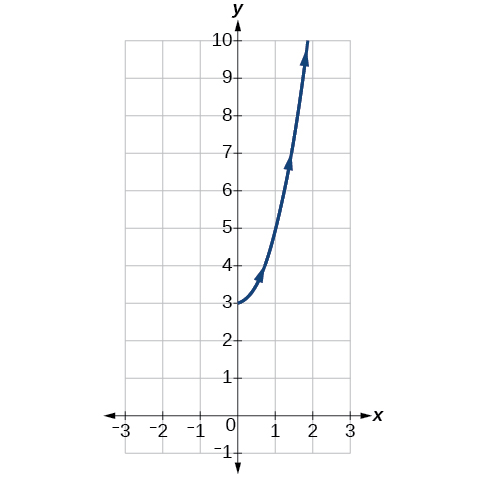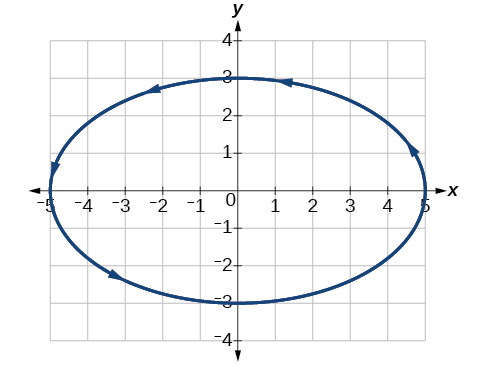# 10.7 Parametric equations: graphs

 Page 1 / 4
In this section you will:
• Graph plane curves described by parametric equations by plotting points.
• Graph parametric equations.

It is the bottom of the ninth inning, with two outs and two men on base. The home team is losing by two runs. The batter swings and hits the baseball at 140 feet per second and at an angle of approximately $\text{\hspace{0.17em}}45°\text{\hspace{0.17em}}$ to the horizontal. How far will the ball travel? Will it clear the fence for a game-winning home run? The outcome may depend partly on other factors (for example, the wind), but mathematicians can model the path of a projectile and predict approximately how far it will travel using parametric equations . In this section, we’ll discuss parametric equations and some common applications, such as projectile motion problems.Parametric equations can model the path of a projectile. (credit: Paul Kreher, Flickr)

## Graphing parametric equations by plotting points

In lieu of a graphing calculator or a computer graphing program, plotting points to represent the graph of an equation is the standard method. As long as we are careful in calculating the values, point-plotting is highly dependable.

Given a pair of parametric equations, sketch a graph by plotting points.

1. Construct a table with three columns: $\text{\hspace{0.17em}}t,x\left(t\right),\text{and}\text{\hspace{0.17em}}\text{\hspace{0.17em}}y\left(t\right).$
2. Evaluate $x$ and $y$ for values of $t$ over the interval for which the functions are defined.
3. Plot the resulting pairs $\text{\hspace{0.17em}}\left(x,y\right).$

## Sketching the graph of a pair of parametric equations by plotting points

Sketch the graph of the parametric equations $x\left(t\right)={t}^{2}+1,\text{\hspace{0.17em}}\text{\hspace{0.17em}}y\left(t\right)=2+t.$

Construct a table of values for $\text{\hspace{0.17em}}t,x\left(t\right),\text{\hspace{0.17em}}$ and $\text{\hspace{0.17em}}y\left(t\right),\text{\hspace{0.17em}}$ as in [link] , and plot the points in a plane.

$t$ $x\left(t\right)={t}^{2}+1$ $y\left(t\right)=2+t$
$-5$ $26$ $-3$
$-4$ $17$ $-2$
$-3$ $10$ $-1$
$-2$ $5$ $0$
$-1$ $2$ $1$
$0$ $1$ $2$
$1$ $2$ $3$
$2$ $5$ $4$
$3$ $10$ $5$
$4$ $17$ $6$
$5$ $26$ $7$

The graph is a parabola with vertex at the point $\text{\hspace{0.17em}}\left(1,2\right),$ opening to the right. See [link] .

Sketch the graph of the parametric equations $\text{\hspace{0.17em}}x=\sqrt{t},\text{\hspace{0.17em}}\text{\hspace{0.17em}}y=2t+3,\text{\hspace{0.17em}}\text{\hspace{0.17em}}\text{\hspace{0.17em}}0\le t\le 3.$## Sketching the graph of trigonometric parametric equations

Construct a table of values for the given parametric equations and sketch the graph:

$\begin{array}{l}\\ \begin{array}{l}x=2\mathrm{cos}\text{\hspace{0.17em}}t\hfill \\ y=4\mathrm{sin}\text{\hspace{0.17em}}t\hfill \end{array}\end{array}$

Construct a table like that in [link] using angle measure in radians as inputs for $\text{\hspace{0.17em}}t,\text{\hspace{0.17em}}$ and evaluating $\text{\hspace{0.17em}}x\text{\hspace{0.17em}}$ and $\text{\hspace{0.17em}}y.\text{\hspace{0.17em}}$ Using angles with known sine and cosine values for $\text{\hspace{0.17em}}t\text{\hspace{0.17em}}$ makes calculations easier.

$t$ $x=2\mathrm{cos}\text{\hspace{0.17em}}t$ $y=4\mathrm{sin}\text{\hspace{0.17em}}t$
0 $x=2\mathrm{cos}\left(0\right)=2$ $y=4\mathrm{sin}\left(0\right)=0$
$\frac{\pi }{6}$ $x=2\mathrm{cos}\left(\frac{\pi }{6}\right)=\sqrt{3}$ $y=4\mathrm{sin}\left(\frac{\pi }{6}\right)=2$
$\frac{\pi }{3}$ $x=2\mathrm{cos}\left(\frac{\pi }{3}\right)=1$ $y=4\mathrm{sin}\left(\frac{\pi }{3}\right)=2\sqrt{3}$
$\frac{\pi }{2}$ $x=2\mathrm{cos}\left(\frac{\pi }{2}\right)=0$ $y=4\mathrm{sin}\left(\frac{\pi }{2}\right)=4$
$\frac{2\pi }{3}$ $x=2\mathrm{cos}\left(\frac{2\pi }{3}\right)=-1$ $y=4\mathrm{sin}\left(\frac{2\pi }{3}\right)=2\sqrt{3}$
$\frac{5\pi }{6}$ $x=2\mathrm{cos}\left(\frac{5\pi }{6}\right)=-\sqrt{3}$ $y=4\mathrm{sin}\left(\frac{5\pi }{6}\right)=2$
$\pi$ $x=2\mathrm{cos}\left(\pi \right)=-2$ $y=4\mathrm{sin}\left(\pi \right)=0$
$\frac{7\pi }{6}$ $x=2\mathrm{cos}\left(\frac{7\pi }{6}\right)=-\sqrt{3}$ $y=4\mathrm{sin}\left(\frac{7\pi }{6}\right)=-2$
$\frac{4\pi }{3}$ $x=2\mathrm{cos}\left(\frac{4\pi }{3}\right)=-1$ $y=4\mathrm{sin}\left(\frac{4\pi }{3}\right)=-2\sqrt{3}$
$\frac{3\pi }{2}$ $x=2\mathrm{cos}\left(\frac{3\pi }{2}\right)=0$ $y=4\mathrm{sin}\left(\frac{3\pi }{2}\right)=-4$
$\frac{5\pi }{3}$ $x=2\mathrm{cos}\left(\frac{5\pi }{3}\right)=1$ $y=4\mathrm{sin}\left(\frac{5\pi }{3}\right)=-2\sqrt{3}$
$\frac{11\pi }{6}$ $x=2\mathrm{cos}\left(\frac{11\pi }{6}\right)=\sqrt{3}$ $y=4\mathrm{sin}\left(\frac{11\pi }{6}\right)=-2$
$2\pi$ $x=2\mathrm{cos}\left(2\pi \right)=2$ $y=4\mathrm{sin}\left(2\pi \right)=0$

By the symmetry shown in the values of $x$ and $\text{\hspace{0.17em}}y,\text{\hspace{0.17em}}$ we see that the parametric equations represent an ellipse . The ellipse is mapped in a counterclockwise direction as shown by the arrows indicating increasing $\text{\hspace{0.17em}}t\text{\hspace{0.17em}}$ values.

Graph the parametric equations: $\text{\hspace{0.17em}}x=5\mathrm{cos}\text{\hspace{0.17em}}t,\text{\hspace{0.17em}}\text{\hspace{0.17em}}y=3\mathrm{sin}\text{\hspace{0.17em}}t.$## Graphing parametric equations and rectangular form together

Graph the parametric equations $\text{\hspace{0.17em}}x=5\mathrm{cos}\text{\hspace{0.17em}}t\text{\hspace{0.17em}}$ and $\text{\hspace{0.17em}}y=2\mathrm{sin}\text{\hspace{0.17em}}t.\text{\hspace{0.17em}}$ First, construct the graph using data points generated from the parametric form . Then graph the rectangular form of the equation. Compare the two graphs.

Construct a table of values like that in [link] .

$t$ $x=5\mathrm{cos}\text{\hspace{0.17em}}t$ $y=2\mathrm{sin}\text{\hspace{0.17em}}t$
$\text{0}$ $x=5\mathrm{cos}\left(0\right)=5$ $y=2\mathrm{sin}\left(0\right)=0$
$\text{1}$ $x=5\mathrm{cos}\left(1\right)\approx 2.7$ $y=2\mathrm{sin}\left(1\right)\approx 1.7$
$\text{2}$ $x=5\mathrm{cos}\left(2\right)\approx -2.1$ $y=2\mathrm{sin}\left(2\right)\approx 1.8$
$\text{3}$ $x=5\mathrm{cos}\left(3\right)\approx -4.95$ $y=2\mathrm{sin}\left(3\right)\approx 0.28$
$\text{4}$ $x=5\mathrm{cos}\left(4\right)\approx -3.3$ $y=2\mathrm{sin}\left(4\right)\approx -1.5$
$\text{5}$ $x=5\mathrm{cos}\left(5\right)\approx 1.4$ $y=2\mathrm{sin}\left(5\right)\approx -1.9$
$-1$ $x=5\mathrm{cos}\left(-1\right)\approx 2.7$ $y=2\mathrm{sin}\left(-1\right)\approx -1.7$
$-2$ $x=5\mathrm{cos}\left(-2\right)\approx -2.1$ $y=2\mathrm{sin}\left(-2\right)\approx -1.8$
$-3$ $x=5\mathrm{cos}\left(-3\right)\approx -4.95$ $y=2\mathrm{sin}\left(-3\right)\approx -0.28$
$-4$ $x=5\mathrm{cos}\left(-4\right)\approx -3.3$ $y=2\mathrm{sin}\left(-4\right)\approx 1.5$
$-5$ $x=5\mathrm{cos}\left(-5\right)\approx 1.4$ $y=2\mathrm{sin}\left(-5\right)\approx 1.9$

Plot the $\text{\hspace{0.17em}}\left(x,y\right)\text{\hspace{0.17em}}$ values from the table. See [link] .

Next, translate the parametric equations to rectangular form. To do this, we solve for $\text{\hspace{0.17em}}t\text{\hspace{0.17em}}$ in either $\text{\hspace{0.17em}}x\left(t\right)\text{\hspace{0.17em}}$ or $\text{\hspace{0.17em}}y\left(t\right),\text{\hspace{0.17em}}$ and then substitute the expression for $\text{\hspace{0.17em}}t\text{\hspace{0.17em}}$ in the other equation. The result will be a function $y\left(x\right)$ if solving for $\text{\hspace{0.17em}}t\text{\hspace{0.17em}}$ as a function of $\text{\hspace{0.17em}}x,\text{\hspace{0.17em}}$ or $\text{\hspace{0.17em}}x\left(y\right)$ if solving for $\text{\hspace{0.17em}}t\text{\hspace{0.17em}}$ as a function of $\text{\hspace{0.17em}}y.\text{\hspace{0.17em}}$

Then, use the Pythagorean Theorem    .

$\begin{array}{r}\hfill {\mathrm{cos}}^{2}t+{\mathrm{sin}}^{2}t=1\\ \hfill {\left(\frac{x}{5}\right)}^{2}+{\left(\frac{y}{2}\right)}^{2}=1\\ \hfill \frac{{x}^{2}}{25}+\frac{{y}^{2}}{4}=1\end{array}$

bsc F. y algebra and trigonometry pepper 2
given that x= 3/5 find sin 3x
4
DB
remove any signs and collect terms of -2(8a-3b-c)
-16a+6b+2c
Will
Joeval
(x2-2x+8)-4(x2-3x+5)
sorry
Miranda
x²-2x+9-4x²+12x-20 -3x²+10x+11
Miranda
x²-2x+9-4x²+12x-20 -3x²+10x+11
Miranda
(X2-2X+8)-4(X2-3X+5)=0 ?
master
The anwser is imaginary number if you want to know The anwser of the expression you must arrange The expression and use quadratic formula To find the answer
master
The anwser is imaginary number if you want to know The anwser of the expression you must arrange The expression and use quadratic formula To find the answer
master
Y
master
master
Soo sorry (5±Root11* i)/3
master
Mukhtar
explain and give four example of hyperbolic function
What is the correct rational algebraic expression of the given "a fraction whose denominator is 10 more than the numerator y?
y/y+10
Mr
Find nth derivative of eax sin (bx + c).
Find area common to the parabola y2 = 4ax and x2 = 4ay.
Anurag
A rectangular garden is 25ft wide. if its area is 1125ft, what is the length of the garden
to find the length I divide the area by the wide wich means 1125ft/25ft=45
Miranda
thanks
Jhovie
What do you call a relation where each element in the domain is related to only one value in the range by some rules?
A banana.
Yaona
given 4cot thither +3=0and 0°<thither <180° use a sketch to determine the value of the following a)cos thither
what are you up to?
nothing up todat yet
Miranda
hi
jai
hello
jai
Miranda Drice
jai
aap konsi country se ho
jai
which language is that
Miranda
I am living in india
jai
good
Miranda
what is the formula for calculating algebraic
I think the formula for calculating algebraic is the statement of the equality of two expression stimulate by a set of addition, multiplication, soustraction, division, raising to a power and extraction of Root. U believe by having those in the equation you will be in measure to calculate it
Miranda
state and prove Cayley hamilton therom
hello
Propessor
hi
Miranda
the Cayley hamilton Theorem state if A is a square matrix and if f(x) is its characterics polynomial then f(x)=0 in another ways evey square matrix is a root of its chatacteristics polynomial.
Miranda
hi
jai
hi Miranda
jai
thanks
Propessor
welcome
jai
What is algebra
algebra is a branch of the mathematics to calculate expressions follow.
Miranda
Miranda Drice would you mind teaching me mathematics? I think you are really good at math. I'm not good at it. In fact I hate it. 😅😅😅
Jeffrey
lolll who told you I'm good at it
Miranda
something seems to wispher me to my ear that u are good at it. lol
Jeffrey
lolllll if you say so
Miranda
but seriously, Im really bad at math. And I hate it. But you see, I downloaded this app two months ago hoping to master it.
Jeffrey
which grade are you in though
Miranda
oh woww I understand
Miranda
Jeffrey
Jeffrey
Miranda
how come you finished in college and you don't like math though
Miranda
gotta practice, holmie
Steve
if you never use it you won't be able to appreciate it
Steve
I don't know why. But Im trying to like it.
Jeffrey
yes steve. you're right
Jeffrey
so you better
Miranda
what is the solution of the given equation?
which equation
Miranda
I dont know. lol
Jeffrey
Miranda
JeffreyByBy OpenStaxBy Richley CrapoBy OpenStaxBy Laurence BailenBy Cath YuBy Anindyo MukhopadhyayByByBy Katy KeilersBy Madison Christian